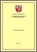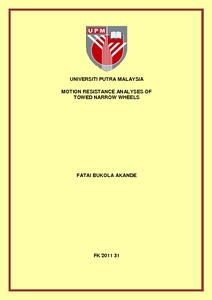Simple Search:

# Motion resistance analyses of towed narrow wheels

## Citation

Akande, Fatai Bukola (2011) Motion resistance analyses of towed narrow wheels. PhD thesis, Universiti Putra Malaysia.

## Abstract / Synopsis

The off and on-road performances of the narrow wheels with respect to the motion resistance and the motion resistance ratio were investigated to obtain design information for the development of simple and low cost agricultural machinery with narrow wheels as traction members.A single wheel motion resistance test rig for towed narrow wheel was developed. The motion resistance was measured in real time by the use of Mecmesin Basic Force Gauge (BFG 2500) interfaced with the notebook with the Dataplot program installed for the display of the real time data acquisition from the BFG. Motion resistance data obtained through this method were called empirical data. While motion resistance ratios predicted from the analytical method using existing mobility and motion resistance ratio models were called analytical data. These analytical data were compared with those obtained empirically under various test conditions on the tilled and wet surfaces. The motion resistance and motion resistance ratios of 660 mm, 610 mm, 510 mm and 405 mm pneumatic bicycle wheels were determined on paved surface, grass field, tilled and the wet surfaces. The other test variables were the dynamic loads at four levels 98.1 N, 196.2 N, 392.4 N and 588.6 N, and the tyre inflation pressures of 276 kPa, 345 kPa and 414 kPa, towing velocity; 4.44 km/h, 6.3 km/hr and 8.28km/h. The 660 mm wheel have the lowest occurring motion resistance, and a rigid wheel of the same rim diameter was constructed and its motion resistance was investigated on the four test surfaces with the same test variables (dynamic loads and towing velocity) as the 660mm pneumatic wheel. The effects of the overall wheel diameter, tyre inflation pressures, dynamic loads and towing velocity on the motion resistance ratio were investigated different test surfaces using regression analysis. The Analyses of variance was carried out at 95% confidence level on all the motion resistance measured on various test surfaces and test conditions. The results of the analyses of variance indicate that there were significant differences between the means of the motion resistance measured on the four test surfaces and between the means of motion resistance measured at all level of the independent variables. Motion resistance recorded on the wet surface were greater than those obtained on the tilled surface and the least were obtained on the paved surface and the grass field although the motion resistances measured were close in values with those on paved surface leading. The motion resistance ratio is inversely proportional to the overall wheel diameter, similarly, the motion resistance ratio is inversely proportional to the dynamic load but the motion resistance has a direct relationship with the dynamic load on all the test surfaces. However, the motion resistance ratio is directly proportional to the dynamic load on tilled surface. Some of the relationships between the motion resistance ratio and each of the test variables are test surface dependent as the relationship varied from surface to surface in some cases. Relationship between motion resistance ratios and the tyre pressure is inversely proportional as it also depends on the test surface and other variables. The motion resistances of the rigid wheel were greater than the pneumatic wheel on all the test surfaces. The motion resistance ratio is inversely proportional to the towing velocity on paved and grass surfaces for pneumatic wheel and on paved and wet surfaces for the rigid wheel. The rigid wheel is a better traction member on wet surface at all levels of towing velocities. A tyre inflation pressure of 414 kPa is recommended for all test surfaces at all levels of dynamic load. The motion resistance ratios obtained through the empirical method were higher than the analytical data on both the tilled and the wet surfaces. From the empirical data, mathematical models derived for predicting motion resistance ratio from the combination of the test variables show that different regression equations fit the different test surfaces; logarithmic relationship fits the tilled surface, while a full and pure quadratic relationship fits the paved surface and the grass field respectively. Some empirical models have been derived for the prediction of the motion resistance ratios of pneumatic and rigid bicycle wheels from the comparison of data obtained by the two methods.

## Download FilePreview
PDF
FK 2011 31R.pdf

Download (1MB) | Preview

## Additional Metadata

Item Type: Thesis (PhD) Wheels FK 2011 31 Professor Desa Bin Ahmad, PhD, Ir Faculty of Engineering Haridan Mohd Jais 12 Mar 2015 11:18 12 Mar 2015 11:18 http://psasir.upm.edu.my/id/eprint/33943 View Download Statistic

### Actions (login required)View Item